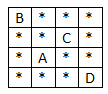# Find alphabet in a Matrix which has maximum number of stars around it in C++

Suppose we have a matrix M. This is filled with stars and letters. We have to find which letter has maximum number of stars around it. So if the matrix is like below −Here A and C has 7 stars around it. this is maximum. As A is lexicographically smaller, so it will be the output.

The approach is simple, we will count the characters, then when one character has found, then count the stars around it. Also store the value inside a map. From the map where the size is maximum that will be printed.

## Example

Live Demo

#include <iostream>
#include<unordered_map>
#define MAX 4
using namespace std;
int checkStarCount(int mat[][MAX], int i, int j, int n) {
int count = 0;
int move_row[] = { -1, -1, -1, 0, 0, 1, 1, 1 };
int move_col[] = { -1, 0, 1, -1, 1, -1, 0, 1 };
for (int k = 0; k < 8; k++) {
int x = i + move_row[k];
int y = j + move_col[k];
if (x >= 0 && x < n && y >= 0 && y < n && mat[x][y] == '*')
count++;
}
return count;
}
char charWithMaxStar(int mat[], int n) {
unordered_map<char, int> star_count_map;
for (int i = 0; i < n; i++) {
for (int j = 0; j < n; j++) {
if ((mat[i][j] - 'A') >= 0 && (mat[i][j] - 'A') < 26) {
int stars = checkStarCount(mat, i, j, n);
star_count_map[mat[i][j]] = stars;
}
}
}
int max = -1;
char result = 'Z' + 1;
for (auto x : star_count_map) {
if (x.second > max || (x.second == max && x.first < result)) {
max = x.second;
result = x.first;
}
}
return result;
}
int main() {
int mat[] = {
{ 'B', '*', '*', '*' },
{ '*', '*', 'C', '*' },
{ '*', 'A', '*', '*' },
{ '*', '*', '*', 'D' }
};
int n = 4;
cout << charWithMaxStar(mat, n) << " has maximum amount of stars around it";
}



### Output

A has maximum amount of stars around it